I have another doubt regarding cvx solution.

I have given everything in CVX format , but it is giving as Failed, but in some cases, it is satisfied with one constraint and doesn’t satisfied with the another constraint.

And cvx is running for one iteration only eventhough, I have given 10 iterations. Plz help me in this regard.

Please post the program, preferably complete with all input data, using Preformatted text icon. And show all CVX and solver output (which means you should NOT use the quiet option), together with whatever other evidence of something occurring which you believe is incorrect or don’t understand/

You should look at the CVX Status to see why it failed. For instance, due to being infeasible. A problem might be feasible with one set of constraints; and if one of the constraints is changed to a different constraint, could be infeasible. All but section 1 of Debugging infeasible models - YALMIP also applies to debugging infeasible problems in CVX.

if you try to solve a sequence of CVX problems in which the results of one problem are used as input data t the next problem, for instance in Successive Convex Approximation, it may be that the initial CVX problem is solved to optimality, but a subsequent problem becomes infeasible, or perhaps the solver fails due to numerical issues, which would happen if the results and hence input data eventually become too extreme for the solver to handle.

Note that if a problem is found to be infeasible., CVX populates all the CVX variables with NaN. If those NaN values are used to generate input data for the next CVX problem, there will be some type of failure, because CVX can’t handle NaN data, because it doesn’t follow CVX’s DCP rules.

Have you proven that is a convex optimization problem? You are violating the DCP rule which requires the argument of norm to be concave.

help inv_pos

 inv_pos   Reciprocal of a positive quantity.
inv_pos(X) returns 1./X if X is positive, and +Inf otherwise.
X must be real.

For matrices and N-D arrays, the function is applied to each element.

Disciplined convex programming information:
inv_pos is convex and nonincreasing; therefore, when used in CVX
specifications, its argument must be concave (or affine).


The problem is convex optimization problem only Sir and

f_theta = f + a_u*diag(h)phase_initial;
f_theta_opt = f + a_u
diag(h)*phase_shift;
and then

obj_6 = (f_theta’*f_theta )*inv_pos(norm( f_theta_opt, 2));
Here f_theta contains optimization variable ‘’ phase_shift"

As per my understanding,norm( f_theta_opt, 2) will always be real as it is norm , hence inv_pos(X), where X is real only.
But is giving error in : Error in cvx/inv_pos (line 5)
y = pow_cvx( x, -1, ‘pow_p’ ); I think error occurs when calculating norm(f_theta_opt)

The original problem is given as: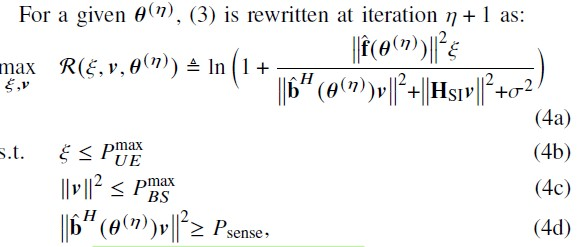Here, the objective function is non-concave and constraint (4d) is non-convex also, which is difficult to solve.

Hence, it has been approximated with the concave lower bound And it is given as follows: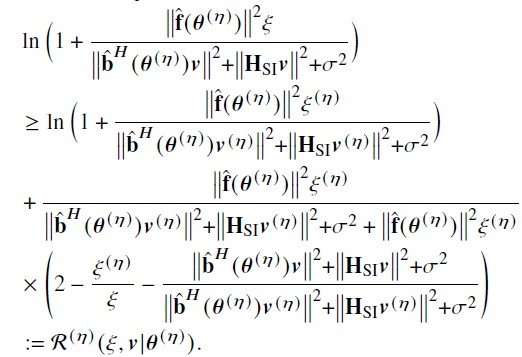Now, the constraints (4b) and (4c) are convex only. But constraint (4d) is a non-convex constraint, which can be approximated as follows: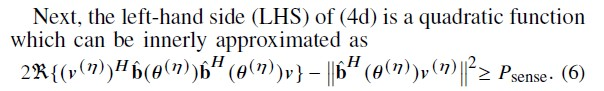Now, the final problem is given as follows: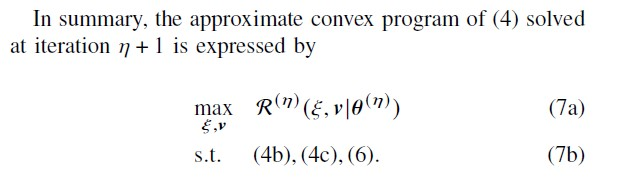If you observe from the above final problem, it is very clear regarding the following conclusions:

max {Concave}
s.t. convex constraints

Hence, I can say that given problem is convex optimization problem Sir.

It would be great help if you look into my original code according to the final optimization problem, Which I am struggling so much Sir.

It appears that your difficulty is associated with the (supposedly) concave lower bound used as the objective function in lieu of the original objective function. I suppose all the things with superscript (\eta) are updated on each “outer” iteration, and constitute input data for each CVX problem you wish to solve.

if that is correct, then:

Why even bother putting the first term (the term with the log) in the objective function, because it is constant?

Presuming \zeta^(n) > 0 which It will be if you use inv_pos as per below , the other term looks like it is easily dealt with; but your code seems to bear little relation to it. You can use
stuff_1*(2 - zeta_n*inv_pos(zeta) -(square_pos(norm(b_tilde'*theta_n*v)) + stuff_2)/stuff_3)

I have no idea why your code has inv_pos(norm(variable)), which generated the error message. I haven’t checked the rest of your code, but given the errors you have made, I would not assume you have not made other mistakes in implementing the formulation in the images.

Presuming you implement this correctly (per the paper or book the images are from), and without my having seen that paper or book, I have a skeptical viiew as to whether this algorithm will work well, or at all, at least depending on the input data and starting values used. Perhaps it worked for the example(s) in that paper or book, but even if so, that doesn’t mean it will wok on other problem instances. But that’s a matter for you (and your advisor?) to worry about.

It is working fine when zeta_n is scalar, but problem arises when it is vector as I mentioned in my previous code

I don’t see any indication anywhere that zeta or zeta_n are not scalars. If your zeta_n is not a scalar, perhaps you are trying to do something which doesn’t make sense?

If one or both of zeta and zeta_n are vectors, the first thing for you to do is to figure out what the mathematics is supposed to be; then you can worry about the code to implement it. The objective function must evaluate to a real scalar.

I suggest you slow down, re-read the paper or book very carefully, and make sure you understand every nuance of the mathematics of every formulation and reformulation. That certainly includes, but is not limited to, understanding the dimensions of everything, and what are input data vs. optimization variables for every optimization problem to be solved. Then figure out how to implement it.

Respected Sir,

Here zeta_n = P_UE_initial and zeta = P_UE (optimization variable)

Now, the expression given as :

obj_3 = ( 2 - (P_UE_initialinv_pos(P_UE)) - ( ( P_BSnorm(b_thetav_mrt1, 2) + P_BSnorm(h_s_iv_mrt1, 2) + sigma_sqr) /(beta_initialnorm(b_thetav_mrt1, 2) + beta_initialnorm(h_s_i*v_mrt1, 2) + sigma_sqr) ) );

Which is working fine and no errors at " (P_UE_initial*inv_pos(P_UE))".

But when

obj_6 = ( (f_theta’*f_theta) *inv_pos(f_theta_opt’*f_theta_opt));
Where

       f_theta = f + a_u*diag(h)*phase_initial;
f_theta_opt = f + a_u*diag(h)*phase_shift; (Which contains optimization variable), then  obj_6 = ( (f_theta'*f_theta) *inv_pos(f_theta_opt'*f_theta_opt)); giving error as


Error using cvx/pow_cvx
Disciplined convex programming
error:
Illegal operation: pow_p(
{convex}, {-1} )

Error in cvx/inv_pos (line 5)
y = pow_cvx( x, -1, ‘pow_p’ );

Error in RIS_FD_ISAC_Optimization (line 470)
obj_6 = ( (f_theta’*f_theta) *inv_pos(f_theta_opt’*f_theta_opt));

As you said f_theta’*f_theta and f_theta_opt’*f_theta_opt are scalars only as those are resultants of norm(x,2) but second one contains optimization variable which is giving error related to pow{} like that .
There I am getting error Sir.

I have already send you the image related to it: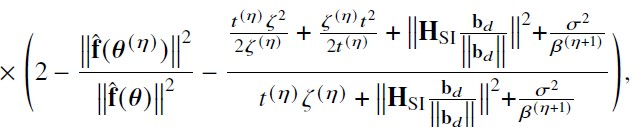I have no idea what you are doing. As I told you before, the argument of inv_pos is not allowed to be convex. But I don’t know why you are trying to call inv_pos with a convex argument.

I don’t see what zeta or zeta_n should be vectors. I believe zeta_n should be a constant, which is initialized before the first CVX problem, and then updated with the optimal value from CVX for use in the next call to CVX problem.

In any event, your series of posts is a confusing mess.I need to see a complete code, with the error messages from executing it if I am to provide more help.

Have you followed the advice from my previous post? I suggest you do so before posting again.

I suggest you slow down, re-read the paper or book very carefully, and make sure you understand every nuance of the mathematics of every formulation and reformulation. That certainly includes, but is not limited to, understanding the dimensions of everything, and what are input data vs. optimization variables for every optimization problem to be solved. Then figure out how to implement it.

I still have no idea why your code has inv_pos(norm(...)). I fail to see how such expression belongs in your model, leaving aside consideration of whether it is DC-compliant.

If you “arbitrarily” insert a minus sign somewhere, and it is not a correction of an erroneous omission of a minus sign which belongs there, that indicates your code is not based on a careful implementation of anything. And of course that is a different model. t is apparent you have no yet achieved a sufficient understanding of what you are doing.

Sir, I have posted so many times regarding inv_pos, still you are asking. Ok, No problem. I am providing again here:Denomonator term having that norm{f(theta),2} which includes an optimization variable phase_shift. That is the reason I am writing that inv_pos{norm(f,2)}. But What I observed that the term (1./ convex ) is cvx is not accepting hence it is giving error as " Illegal operation: pow_p(
{convex}, {-1} ) "

Now, I am in the process of modifying it. For this I have done 2+ remaining stuff. Now, No errors but result is infeasible with +Inf optimal value Sir.

But overall objective function is concave only Sir

You keep changing and showing different stuff. That makes things difficult for me. Are you juming around between different expressions and formulations in the paper, and perhaps they don’t all constitute convex optimization problems?

I have been looking at the expression for R^{(n)}. That has \zeta in the denominator, for which you can use inv_pos. I don’t see any \theta as an optimization variable. There is \theta^{(n)}, inside a norm, but all the items in that norm should be input data, so that norm is not a CVX expression.

It seems you have not seen my code properly Sir.

You are correct for zeta and is it is sub-problem-1 . No issues here.

Coming to sub-problem-2, here theta as an optimization variable. For you, I have provided only one sub-problem to understand .
I think you got my point.

Objective function is concave and constraints are convex. Hence, I can solve using cvx with max{concave} s.t. convex constraints.

Is it clear sir.

I need help at sub-problem 2 only. I think you understood my question now.

And with subproblem-1 , No errors and program runs but giving infeasible (one constraint is satisfied , other one is not satisfied). I think cvx unable to solve??? Should I move to another platform? Plz tell this Sir